# Electrical calculations

All the calculation factors given below are informations without commitment of any kind on our part. You can make your own estimates, ask us for advice (note: we will only engage in a study if it is part of a precise study contract signed between the 2 parties), or ask a design office.

 Heating of liquids, gases or solids (For the calculation of a mass to heat) Heat loss of a surface (For calculations of insulation or temperature containment) Heat transfer by conduction (For calculations of insulation or temperature containment) plane / cylindrical surface Heat transfer by radiating (For calculations of power losses or entry) Heat exchange by radiation between 2 surfaces (Heating by radiating) Heating by convection State change (fusion and vaporization)

## Heating of liquids, gases or solids

(for the calculation of a mass to heat)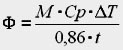where :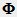= power in Watt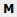= mass to heat in kg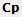= specific heat in kcal/kg.K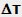= temperature rise in °C ou K

0,86 = coeff. of conversion kcal/h to Watt
t = temperature rise duration in hours

## Heating loss of a surface (natural convection)

(for calculations of insulation or temperature containment)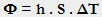where := power in Watt
h   = Coeff. of convection in W/m²
= surface area in m²= temperature difference between the surface and the air in °C or K

### Heat loss by natural convection

Heating surface chauffante in ambiant air following the simplified formula according to Mac Adams.= temperature difference between the heating surface chauffante and ambiant air
h1 = Coefficient of convection of a horizontal plate, heating face facing up
h2 = Coefficient of convection of a horizontal plate, heating face facing down
h3 =  Coefficient of convection of a vertical plate more than 0.3m high
h4 = Coefficient of convection of a vertical plate less than 0.3m high

Table of dissipated power by convection in Watts/m² in function of the DT and the type of surface: :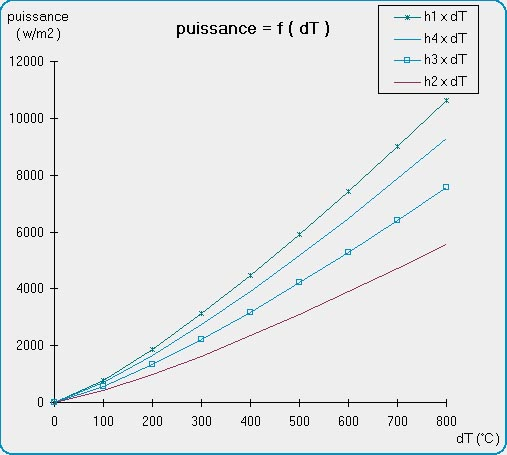## Heat transfer by conduction

(for example for the calculation of heat losses through a wall)

Plane wall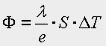Cylindrical wall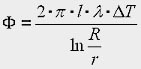where := power in Watt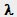= Coeff. of conduction of materials in W/m.K
S  = surface area of materials in m²= temperature difference between the 2 walls in °C ou K
= length of cylindrical wall in m
R and r = interiot and exterior radius and the wall in m.

(For the calculation of power losses or entry)

Generalized Kirchhoff law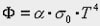where := power in Watt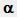= absorption or emission factor of emitting surface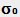= 5,675 .  10-8  W/m².K4
T = absolute temperature in K.

## Heat exchange by radiating between 2 surfaces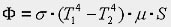where := power in Watt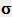= mutual coeff. of radiating between the 2 surfaces W/m².K4
T1 and T2 = absolute temperature of the 2 surfaces in K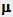= angle factor d’angle or surfaces’ shape factor

## Heating by convection

There are many cases of convective heat transfer.
It may be natural (as in radiators or loss of a wall) see above, or forced (case heaters, unit heaters, etc …).
In each case we must consider the shape and size as well as laminar or turbulent flow.

## State change

(fusion or evaporation)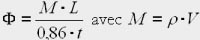where := power in Watt
M = Weight in kg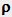= masse density in kg/dm3
= Volume in dm3 (or liter)
= latent heat of fusion or evaporation in kcal/kg.
t = time in hour